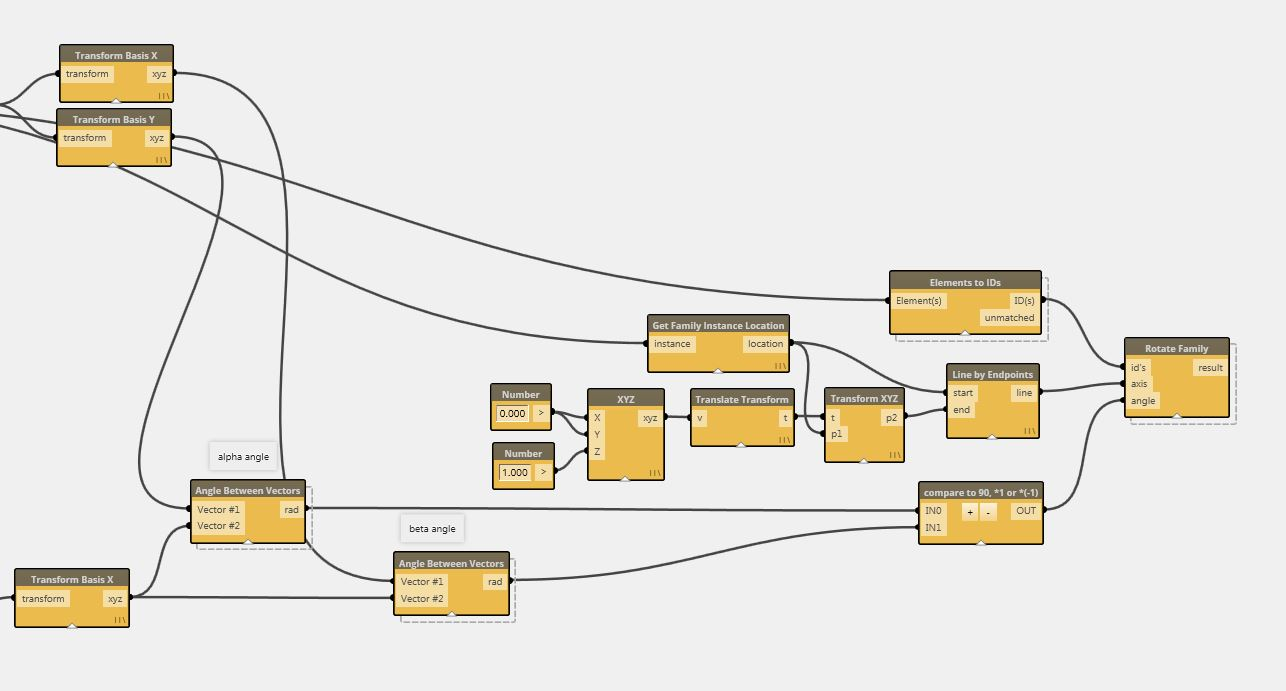# rotate family with dynamo

Below is a quick overview of a method to create an array of family instances on a curve and rotate them to always be perpendicular to host curve.

You will need to download a few packages to get it to work.

1. Family Transform – extracts Transformation Matrix from family instances. Used to determine angle for rotation.

2. Angle Between Vectors – calculates angle in radians between two vectors. used to determine angle for rotation.

3. Element to ID’s – extracts family instance ID. Used with Rotate Family component.

4. Rotate Family – rotates Revit family instance by a given angle (radians) around given axis (line).There is also a component that I am calling “compare to 90, *1 or *(-1)”. What I am doing there is to compare an angle to 90 degrees and if that angle is smaller it gets multiplied by 1 but if its larger i am multiplying it by -1. I am doing this to compensate for the fact that some tangents on a curve are either in the “negative” or “positive” based on whether a curve is concave or convex. However when measuring an angle between curve tangent and any other angle revit will always give you the smallest angle and never in negative degrees. Here’s a python code for that node. I am not publishing it since it’s a bit of a rough/specific application for this example thus not very useful to others:

1.Tamer Salheen says:

I am trying to achieve the family rotation but apparently there are something missing! I appreciate your help in this. I am new to Dynamo and as usual don’t have the sufficient time to dig this properly.
I am trying to build a tunnel model based on given coordinates for the rings. I’ve created a simplified ring family with parameters for rotation and trying ot feedback the rotation angle from Dynamo by retrieving the angle between two vectors. I’m considering the horizontal angle for the moment and I believe if the horizontal is resolved the vertical will be as well. the rotation will be a given information from site as a list so I am not worrying much about it.

••